## RS Aggarwal Class 6 Solutions Chapter 21 Concept of Perimeter and Area Ex 21D

These Solutions are part of RS Aggarwal Solutions Class 6. Here we have given RS Aggarwal Solutions Class 6 Chapter 21 Concept of Perimeter and Area Ex 21D

Other Exercises

Question 1.
Solution:
(i) Length (l) = 46 cm
Area of rectangle = l x b
= 46 x 25 sq. cm
= 1150 sq.cm.
(ii) Length (l) = 9 m
Area = l x b = 9 x 6
= 54 sq. metre Ans.
(iii) Length (l) = 14.5 m
Area = l x b
= 14.5 x 6.8 sq. m
= 98.6 sq. m Ans.
(iv) Length (l) = 2m 5cm
= 2x 100 cm + 5 cm
= 200 cm + 5 cm
= 205 cm
Area = l x b
= 205 cm x 60 cm
= 12300 cm²
(v) Length (l) = 3.5 km
Area = l x b
= 3.5 x 2
= 7 sq. km. Ans.

Question 2.
Solution:
Side of a square plot = 14 m
Area = (Side)²
= (14)²
= 196 m²

Question 3.
Solution:
Length of top of table (l) = 2 m, 25 cm = 2.25 m
Breadth (l) = 1 m 20 cm = 1.20 m
Area of the top of the table = l x b
= (2.25 x 1.20) sq. m
= 2.7 sq. m. Ans.

Question 4.
Solution:
Length of carpet (l) = 30 m 75 cm
= 30.75 m
Breadth (b) = 80 cm = 0.80 m
Area of the carpet = l x b
= (30.75 x 0.80) sq. m
= 24.6 sq. m
Cost of one square metre = Rs. 20
Total cost = 24.6 x 150
= Rs. 3690. Ans

Question 5.
Solution:
Length of the sheet of paper 3 m 24 cm
= 300 cm + 24 cm
= 324 cm
Breadth of the sheet of the paper 1 m 72 cm
= 100 cm + 72 cm
= 172 cm
Area of the sheet of paper = (324 x 172) cm²
Also, area of the piece of paper required for an envelope = (18 x 12) cm².
Number of envelopes that can be made
= $$\\ \frac { 324\times 172 }{ 18\times 12 }$$
= 258

Question 6.
Solution:
Length of room (l) = 12.5 m
Area = l x b
= (12.5 x 8) sq. m
= 100 sq. m
Side of square carpet (a) = 8 m
Area of carpet = a² = (8 x 8) sq. m.
= 64 square metre
Area left without carpet = 100 sq. m – 64 sq. m
= 36 sq. m Ans.

Question 7.
Solution:
Length of the lane = 150 m
Breadth of the lane = 9 m
Area of the lane = (150 x 9) m²
= 1350 m²
Area of the brick = 22.5 cm x 7.5 cm
= 168.75 cm²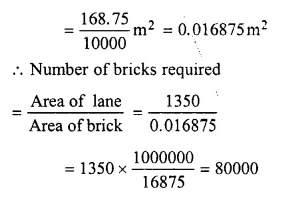Question 8.
Solution:
Length of room (l) = 13 m
Area of floor or carpet = l x b
= 13 x 9
= 117 sq. m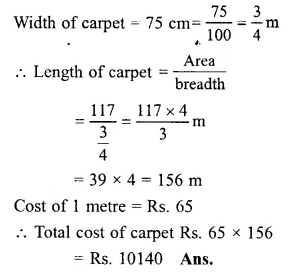Question 9.
Solution:
Let the length of the rectangular park = 5x metres
and the breadth of the rectangular park = 3x metres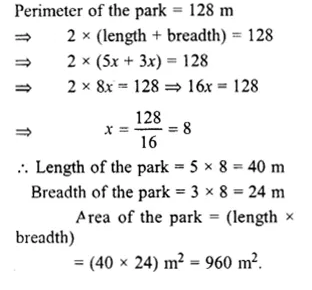Question 10.
Solution:
Side of the square plot = 64 m
Perimeter of the square plot = 4 x Side
= 4 x 64
= 256 m
Perimeter of the rectangular plot = Perimeter of the square plot = 256 m
Length of the rectangular plot = 70 m
Perimeter = 2 x (Length + Breadth)
256 = 2 (70 + b)
256 = 140 + 2b
=> 2b = 256 – 140
=> 2b = 116
b = $$\\ \frac { 116 }{ 2 }$$ = 58 cm
Area of the rectangular plot = (length x breadth)]
= (70 x 58) m²
= 4060 m²
Area of the square plot = Side x Side
= (64 x 64) m²
= 4096 m².
Square plot has the greater area than that of the rectangular plot by
(4096 – 4060)
= 36 m².

Question 11.
Solution:
Total cost of cultivating the rectangular field = Rs. 71400
Rate of cultivating = Rs. 35 per sq. m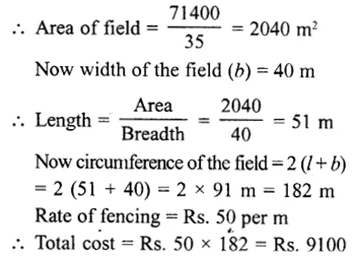Question 12.
Solution:
Area of rectangle = 540 sq. cm
Length (l) = 36 cmQuestion 13.
Solution:
Measure of a marble tile = 12cm x 10cm
Area of wall = 4 m x 3 m
= 12 m²
Area of one marble tile
= 12 x 10
= 120 cm²Question 14.
Solution:
Area of a rectangle = 600 cm²Question 15.
Solution:
diagonal of square = 5 √2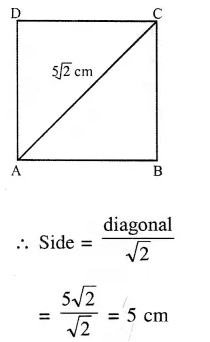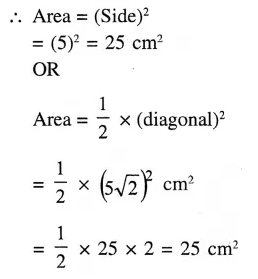Question 16.
Solution:
(i) We name the given region as shown in the figure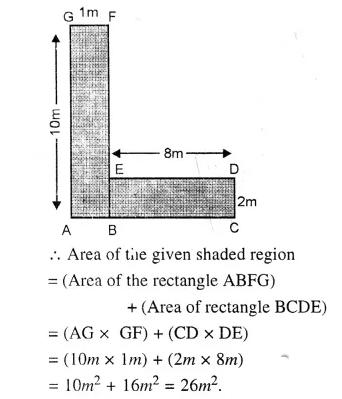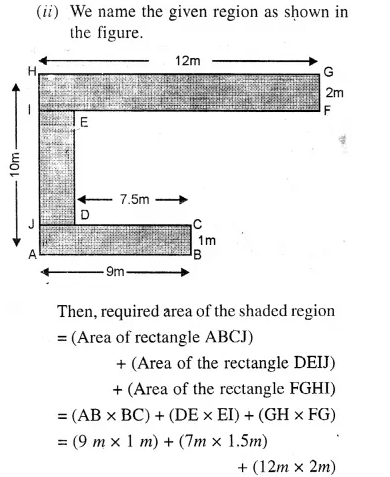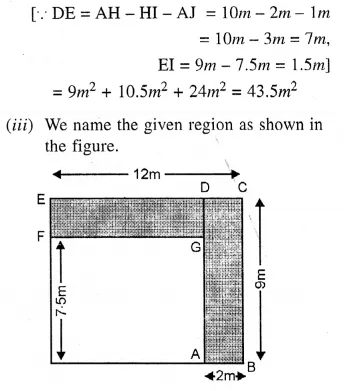Question 17.
Solution:
Measures are in cm
(i) In the figure, there are three rectangles and one square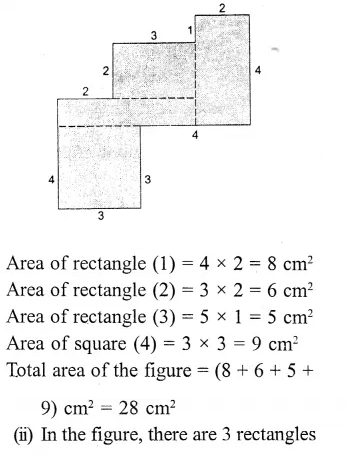= 5(6 x 6) cm²
= 180 cm²

Hope given RS Aggarwal Solutions Class 6 Chapter 21 Concept of Perimeter and Area Ex 21D are helpful to complete your math homework.

If you have any doubts, please comment below. Learn Insta try to provide online math tutoring for you.